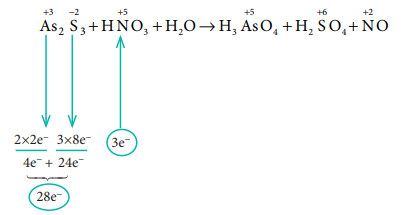Home | | Chemistry 11th std | Evaluate Yourself: Basic Concepts of Chemistry and Chemical Calculations

# Evaluate Yourself: Basic Concepts of Chemistry and Chemical Calculations

Chemistry : Basic Concepts of Chemistry and Chemical Calculations

Evaluate Yourself

1) By applying the knowledge of chemical classification, classify each of the following into elements, compounds or mixtures.

(i) Sugar

(ii) Sea water

(iii) Distilled water

(iv) Carbon dioxide

(v) Copper wire

(vi) Table salt

(vii) Silver plate

(viii) Naphthalene balls

Solution:

(i)  Element - Copper wire, Silver plate

(ii) Compound - Sugar, distilled water, carbon dioxide, Table salt, Naphthalene balls

(iii) Mixture  - Sea water

Evaluate Yourself

2. Calculate the molar mass of the following.

Ethanol(C2H5OH)

Potassium permanganate (KMnO4)

Potassium dichromate (K2Cr2O7)

Sucrose (C12H22O11)

Solution:

(i) C2H5OH:(2 x 12)+ (5 x 1) + (1 x 16) + (1 x 1)=46 g

(ii) KMnO4:(1 x 39)+ (1 x 55) + (4 x 16)=158 g

(iii) K2Cr2O7:(2 x 39)+ (2 x 52) + (7 x 16)=294 g

(iv) C12H22O11:(12 x 12) + (22 x 1) + (11 x 16)=342 g

Evaluate Yourself

3a) Calculate the number of moles present in 9 g of ethane.

3b) Calculate the number of molecules of oxygen gas that occupies a volume of 224 ml at 273 K and 3 atm pressure.

Solution:

(a) Molar mass of ethane, C2H6 = (2 x 12)+ (6 x 1) = 30 g mol-1

n = mass / molar mass = 9 g / 30 g mol-1 = 0.3 mole

(b) At 273 K and 1 atm pressure 1 mole of a gas occupies a volume of 22.4 L

Therefore,

number of moles of oxygen, that occupies a volume of 224 ml at 273 K and 3 atm pressure=0.03 mole

1 mole of oxygen contains 6.022 x 1023 molecules

0.03 mole of oxygen contains = 6.022 x 1023  x 0.03

= 1.807 x 1022 molecules of oxygen

Evaluate Yourself

4a) 0.456 g of a metal gives 0.606 g of its chloride. Calculate the equivalent mass of the metal.

4b) Calculate the equivalent mass of potassium dichromate. The reduction half-reaction in acid medium is,

Cr2O72- + 14H+ +6e- → 2 Cr3+ +7H2O

Solution:

(a) Mass of the metal = 0.456 g

Mass of the metal chloride = 0.606 g

0.456 g of the metal combines with 0.15 g of chlorine.

Mass of the metal that combines with 35.5 g of chlorine is 0.456/0 .15 × 35.5

= 107.92 g eq–1.

(b) Equivalent mass of a oxidising agent =  molar mass / number of moles of electrons gained by one mole of the reducing agent

= 292.2 g mol-1 / 6 eq mol-1

= 48.7 g eq-1

Evaluate Yourself

5) A Compound on analysis gave the following percentage composition C=54.55%, H=9.09%, O=36.36%. Determine the empirical formula of the compound.

Solution:Evaluate Yourself

6) Experimental analysis of a compound containing the elements x,y,z on analysis gave the following data. x = 32 %, y = 24 %, z = 44 %. The relative number of atoms of x, y and z are 2, 1 and 0.5, respectively. (Molecular mass of the compound is 400 g) Find out.

i) The atomic masses of the element x,y,z.

ii) Empirical formula of the compound and

iii) Molecular formula of the compound.

Solution:Calculated empirical formula mass = (16 × 4) + (24 × 2) + 88

= 64 + 48 + 88 = 200Molecular formula (X4Y2Z)2 = X8Y4Z2

Evaluate Yourself

7) The balanced equation for a reaction is given below

2x+3y → 4l + m

When 8 moles of x react with 15 moles of y, then

i) Which is the limiting reagent?

ii) Calculate the amount of products formed.

iii) Calculate the amount of excess reactant left at the end of the reaction.

Solution:Limiting reagent: x

Product formed: 16 moles of l& 4 moles of m

Amount of excess reactant: 3 moles of y

Evaluate Yourself

8) Balance the following equation using oxidation number method

As2S3 + HNO3 + H2O → H3AsO4+H2SO4+NO

Solution:Equate the total no. of electrons in the reactant side by cross multiplying,

3 As2 S3 + 28 HNO3 + H2O → H3AsO4 + H2SO4 + NO

Based on reactant side, balance the products

3 As2 S3 + 28 HNO3 + H2O → 6 H3AsO4 + 9 H2SO4 + 28 NO

Product side:36 hydrogen atoms & 88 oxygen atoms

Reactant side:28 hydrogen atoms & 74 oxygen atoms

Difference is 8 hydrogen atoms & 4 oxygen atoms

Multiply H2O molecule on the reactant side by '4'. Balanced equation is,

3 As2 S3 + 28 HNO3 + 4 H2O → 6 H3AsO4 + 9 H2SO4 + 28 NO

Tags : Solved Example Problems , 11th Chemistry : UNIT 1 : Basic Concepts of Chemistry and Chemical Calculations
Study Material, Lecturing Notes, Assignment, Reference, Wiki description explanation, brief detail
11th Chemistry : UNIT 1 : Basic Concepts of Chemistry and Chemical Calculations : Evaluate Yourself: Basic Concepts of Chemistry and Chemical Calculations | Solved Example Problems